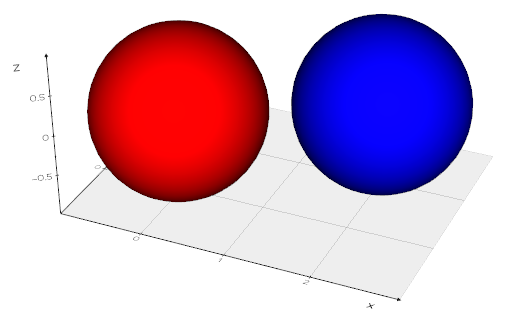# How to label vtkPolyData?

For example, I have two poly data: `poly1 & poly2`. I want to use `vtkAppendPolyData` to merge the two polydata and get `polyFinal`.

Is it possible to label `poly1` and `poly2` before `vtkAppendPolyData` so that I can know the cell in `polyFinal` is belong to `poly1` or `poly2`?

Ang suggestion is appreciated~~~

One idea that comes to mind is to create a 2-tuple cell array with 1)id of polydata, 2)cell id of polydata.

1 Like

something like:

``````...
idarr = []
polyapp = vtk.vtkAppendPolyData()
for i, poly in enumerate(polys):
idarr += [i] * poly.GetNumberOfPoints()
polyapp.Update()
mpoly = polyapp.GetOutput()
varr = numpy2vtk(idarr, dtype=np.uint16, name="OriginalMeshID")
``````

as in:

``````from vedo import *
s1 = Sphere()
s2 = Sphere().shift(2,1,0)
s12 = merge(s1, s2, flag=True)
show(s12, axes=1)
``````Thanks for kindly reply. But I still have some question.

For example, there are 10 points & 20 cells in `poly1`, and 5 points & 10 cells in `poly2`. In this way, there should be 15 points & 30 cells in `mpoly`, which means the merge of `poly1` and `poly2`.

The range of cell id for `poly1` should be 0~19, and the range of cell id for `poly2` should be 0~9. But, for `mpoly`, the range of cell id should be 0~29. For a cell with id 25, how to know whether it belong to `poly1` or `poly2`?

The two `poly` may share some points. In this way, I could not set a unique id for each point. Only the cell is unique.

Is it possible to `AddArray` for cell?

Create a two-component array: the first component is the id of the polydata, the second is the point id.